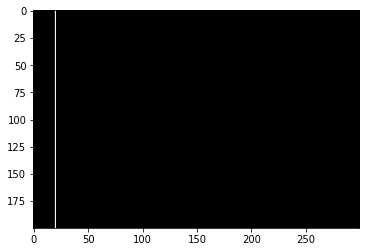# Dimensions of an input image

I am new with Pytorch, and will be glad if someone will be able to help me understand the following (and correct me if I am wrong), regarding the meaning of the command x.view in Pytorch first tutorial:

https://pytorch.org/tutorials/beginner/blitz/cifar10_tutorial.html

( def forward(self, x):
x = self.pool(F.relu(self.conv1(x)))
x = self.pool(F.relu(self.conv2(x)))
x = x.view(-1, 16 * 5 * 5)
x = F.relu(self.fc1(x))
x = F.relu(self.fc2(x))
x = self.fc3(x)
return x)

1. As far as I understand, an input 256X256 image to a convolutional layer is inserted in its 2D form (i.e. - a 256X256 matrix, or a 256X256X3 in the case of a color image). Nevertheless, when we insert an image to a fully-connected linear layer, we need to first reshape the 2D image into a 1D vector. Is this why we use the command “x = x.view(-1, 16 * 5 * 5)” before inserting x into the fully connected layers?

2. If the input image x would be 3D (e.g. 256X256X256), would the syntax of the given above “forward” function remain the same?

1 Like

Your explanation is right in general.
Just some minor issues:
In PyTorch, images are represented as `[channels, height, width]`, so a color image would be `[3, 256, 256]`.
During the training you will get batches of images, so your shape in the forward method will get an additional batch dimension at dim0: `[batch_size, channels, height, width]`.

The `.view()` operation gives you a new view on the tensor without copying any data.
This means `view` is cheap to call and you don’t think too much about potential performance issues.
In your example, `x` is reshaped into a `tensor` of shape `16*5*5` in dim1 and all remaining values in dim0. That’s what the `-1` stands for.
In the case of a 256 -channel image, the `view` operation would resume the same, since the spatial dimensions of the image are the same and the channels are defined by the number of kernels of `conv2`.

18 Likes

Thanks a lot ptrbick!

1 Like

Sorry for revisiting this.

But this particular line seems to suggest the order is `n, b, w, h` i.e., width comes before height. Probably a mistake but better to clarify.

I’m not familiar enough with the repository so cannot comment on the correctness or if internal permutations are applied to the tensor.
The standard is `[N, C, H, W]` for channels-last and `[N, H, W, C]` for channels last.
Here is a small code snippet which draws a vertical line in the H dimension at W=20:

``````# create input image
N, C, H, W = 1, 3, 200, 300
x = torch.zeros(N, C, H, W).to(torch.uint8)
# draw line in H dimension at W=30
x[:, :, :, 20] = 255

img = transforms.ToPILImage()(x)
arr = np.array(img)
print(arr.shape)
# (200, 300, 3) # H, W, C
plt.imshow(arr)
``````

Output:Given the output and the dimension of the output image, the format in PyTorch is `[N, C, H, W]` as the default while `arr` uses the channels-last format as `[H, W, C]` (the batch dimension is missing as `imshow` expects a single image).